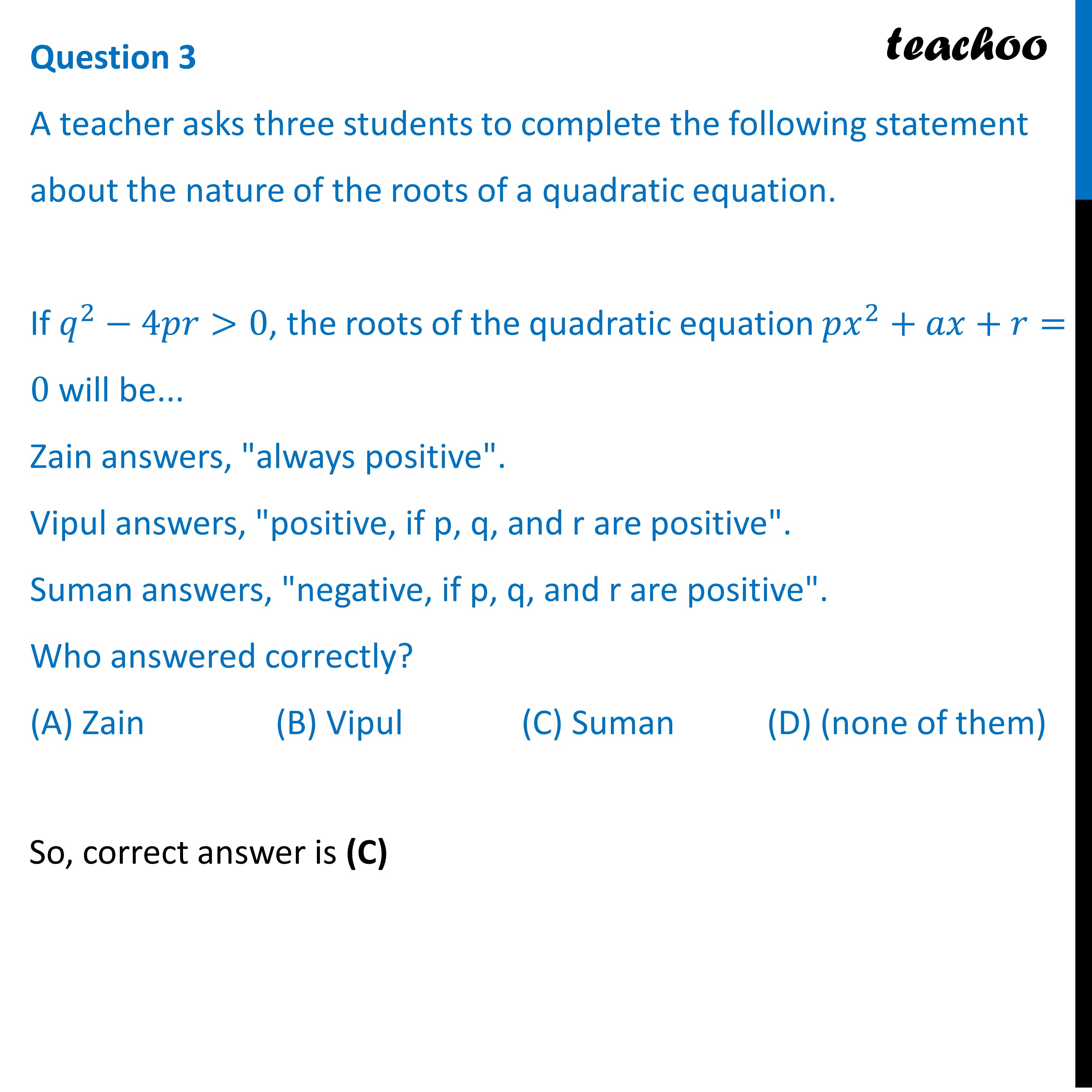Practice Questions CBSE - Maths Class 10 (2023 Boards)

Class 10
Solutions of Sample Papers for Class 10 Boards

## (D) (none of them)Learn in your speed, with individual attention - Teachoo Maths 1-on-1 Class

### Transcript

A teacher asks three students to complete the following statement about the nature of the roots of a quadratic equation. If 𝑞^2−4𝑝𝑟>0, the roots of the quadratic equation 〖𝑝𝑥〗^2+𝑎𝑥+𝑟=0 will be... Zain answers, "always positive". Vipul answers, "positive, if p, q, and r are positive". Suman answers, "negative, if p, q, and r are positive". Who answered correctly? (A) Zain (B) Vipul (C) Suman (D) (none of them)